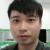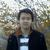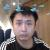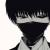提高你的Python: 解释‘yield’和‘Generators（生成器）’已翻译 100%

renwofei423 投递于 2013/04/12 10:57 (共 16 段, 翻译完成于 04-18)

例子：有趣的素数

def get_primes(input_list):
result_list = list()
for element in input_list:
if is_prime(element):
result_list.append()

return result_list

# 或者更好一些的...

def get_primes(input_list):
return (element for element in input_list if is_prime(element))

# 下面是 is_prime 的一种实现...

def is_prime(number):
if number > 1:
if number == 2:
return True
if number % 2 == 0:
return False
for current in range(3, int(math.sqrt(number) + 1), 2):
if number % current == 0:
return False
return True
return False

处理无限序列

def get_primes(start):
for element in magical_infinite_range(start):
if is_prime(element):
return element

def solve_number_10():
# She *is* working on Project Euler #10, I knew it!
total = 2
for next_prime in get_primes(3):
if next_prime < 2000000:
total += next_prime
else:
print(total)
return

走进生成器

(next()会操心如何调用生成器的__next__()方法)。既然生成器是一个迭代器，它可以被用在for循环中。

yield就是专门给生成器用的return(加上点小魔法)。

>>> def simple_generator_function():
>>>    yield 1
>>>    yield 2
>>>    yield 3

>>> for value in simple_generator_function():
>>>     print(value)
1
2
3
>>> our_generator = simple_generator_function()
>>> next(our_generator)
1
>>> next(our_generator)
2
>>> next(our_generator)
3

魔法?

def get_primes(number):
while True:
if is_prime(number):
yield number
number += 1

评论(15)

m
for power in range(iterations)中的iterations是传入一个迭代器吗，要是一个迭代器为啥要加range啊，请赐教，并举一个iterations的示例引用来自“crab2313”的评论引用来自“crab2313”的评论引用来自“crab2313”的评论引用来自“crab2313”的评论引用来自“crab2313”的评论引用来自“crab2313”的评论引用来自“crab2313”的评论引用来自“crab2313”的评论